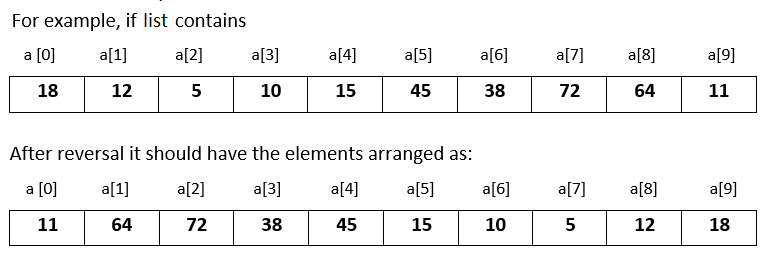# Input 10 numbers in integer list and reverse the original list in Python

###### Lists - Question 7

In this question, we will see how to input 10 numbers in integer list and reverse the original list and print it on the screen in Python programming. To know more about lists click on the lists lesson.

Q7) Write a program in Python to input 10 numbers in integer list and reverse the original list and print it on the screen.#### Program

``````t=0
a=list()

print('Enter 10 numbers')
for i in range(10):
a.append(int(input()))

for i in range(10//2):
t=a[i]
a[i]=a[9-i]
a[9-i]=t

print('\nModified list after reversal')
for n in a:
print(n,end=' ')``````

#### Output

```Enter 10 numbers
18
12
5
10
15
45
38
72
64
11

Modified list after reversal
11 64 72 38 45 15 10 5 12 18```